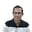Related Tags

golang
sudoku
communitycreator

# How to check a valid solution for the 9x9 fully filled SudokuJeyabalaji Subramanian

### Problem statement

Now that we have a basic understanding of how Goroutines work, let us take a slightly more complex problem and tackle it with Goroutines.

Problem Statement: Given a fully filled 9x9 Sudoku board, find out whether this is a valid solution.

### Solution approach

Whenever we solve problems using concurrency or parallel processing, we first have to come up with the fundamental unit of work that will be called repeatedly. Once we arrive at this core function, everything else is about organizing code around this core function.

Now, what’s the core function that is at play here?

### Core function to validate a row

Once we write this core function, it is just a matter of concurrently passing different slices of a Sudoku boardrows, columns, and boxes to work out a solution.

Let’s look at this core function now.

package main

import (
"fmt"
)

// Structure to hold a row of 9 numbers
type Row int

func (r Row) Valid() bool {

numCountMap := make(map[int]int)

for _, col := range r {
numCountMap[col] = numCountMap[col] + 1

if numCountMap[col] > 1 {
return false
}

if col <= 0 || col > 9 {
return false
}
}

return true
}

func main() {
fmt.Println("Hello, playground")

var myRow Row

myRow = int{1, 2, 3, 4, 9, 5, 6, 7}
fmt.Printf("%v is %t\n", myRow, myRow.Valid())

myRow = int{1, 2, 3, 4, 5, 6, 7, 8, 11}
fmt.Printf("%v is %t\n", myRow, myRow.Valid())

myRow = int{1, 2, 3, 4, 9, 5, 6, 7, 7}
fmt.Printf("%v is %t\n", myRow, myRow.Valid())

myRow = int{1, 2, 3, 4, 5, 6, 7, 8, 9}
fmt.Printf("%v is %t\n", myRow, myRow.Valid())
}


### Sudoku board validation logic

Now that the core function is in place, let’s look at how to use this function to validate a sudoku board.

Each cell in a sudoku board belongs to a row, column, and a bounded box.Sudoku board validation logic:

1. Traverse each cell in a sudoku board and collect this cell into a row, column, and a bounded box.
2. Concurrently pass the rows, columns, and bounded boxes into the core validation function.

Let’s look at a code to see how to perform these steps.

package main

import (
"fmt"
"sync"
"time"
)

var wg sync.WaitGroup

// Row is an integer array of 9 numbers
type Row int

// Sudoku is composed of 9 Rows
type Sudoku Row

// Valid validates whether a Row contains non-repeating numbers 1 to 9
func (r Row) Valid() bool {

defer wg.Done()
numCountMap := make(map[int]int)
var _valid bool

for _, col := range r {
numCountMap[col] = numCountMap[col] + 1

if numCountMap[col] > 1 {
_valid = false
}

if col <= 0 || col > 9 {
_valid = false
}
}

_valid = true

fmt.Printf("%v is %t \n", r, _valid)

return _valid
}

// _getBoundedBoxIndex provides an ID based on row id and column id of a cell
func _getBoundedBoxIndex(rowID int, colID int) int {
bbIndex := 0
switch {
case rowID < 3:
switch {
case colID < 3:
bbIndex = 0
break
case colID < 6:
bbIndex = 1
break
case colID < 9:
bbIndex = 2
break
}
break
case rowID < 6:
switch {
case colID < 3:
bbIndex = 3
break
case colID < 6:
bbIndex = 4
break
case colID < 9:
bbIndex = 5
break
}
break
case rowID < 9:
switch {
case colID < 3:
bbIndex = 6
break
case colID < 6:
bbIndex = 7
break
case colID < 9:
bbIndex = 8
break
}
break
}
return bbIndex
}

// getRow converts a slice into a Row
func getRow(rowSlice []int) Row {
var row Row
for index, col := range rowSlice {
row[index] = col
}

return row

}

// Solved validates whether a sudoku is solved
func (s Sudoku) Validate() {
myColumns := make(map[int][]int)
myBoundedBoxes := make(map[int][]int)

// traverse the Sudoku once to collect Rows, columns and bounded boxes
for rowID, row := range s {

go row.Valid()

for colID, col := range row {

myColumns[colID] = append(myColumns[colID], col)

bbID := _getBoundedBoxIndex(rowID, colID)
myBoundedBoxes[bbID] = append(myBoundedBoxes[bbID], col)
}
}

if len(myColumns) > 0 {
for _, rowSlice := range myColumns {

row := getRow(rowSlice)
go row.Valid()

}
}

if len(myBoundedBoxes) > 0 {
for _, rowSlice := range myBoundedBoxes {

row := getRow(rowSlice)
go row.Valid()

}
}

wg.Wait()

}

func main() {
fmt.Println("Hello, playground")

var mySudoku Sudoku

mySudoku = int{8, 1, 2, 7, 5, 3, 6, 4, 9}
mySudoku = int{9, 4, 3, 6, 8, 2, 1, 7, 5}
mySudoku = int{6, 7, 5, 4, 9, 1, 2, 8, 3}
mySudoku = int{1, 5, 4, 2, 3, 7, 8, 9, 6}
mySudoku = int{3, 6, 9, 8, 4, 5, 7, 2, 1}
mySudoku = int{2, 8, 7, 1, 6, 9, 5, 3, 4}
mySudoku = int{5, 2, 1, 9, 7, 4, 3, 6, 8}
mySudoku = int{4, 3, 8, 5, 2, 6, 9, 1, 7}
mySudoku = int{7, 9, 6, 3, 1, 8, 4, 5, 2}

start := time.Now()
mySudoku.Validate()
elapsed := time.Since(start)
fmt.Printf("Validate took %s", elapsed)
}


RELATED TAGS

golang
sudoku
communitycreator

CONTRIBUTORJeyabalaji Subramanian